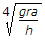# Civil Engineering - Applied Mechanics

### Exercise :: Applied Mechanics - Section 5

51.

The centre of gravity of a quadrant of a circle lies along its central radius at a distance of

 A. 0.2 R B. 0.3 R C. 0.4 R D. 0.5 R E. 0.6 R.

Explanation:

No answer description available for this question. Let us discuss.

52.

A geo-stationary satellite is one which orbits the earth with a velocity of rotation of

 A. moon B. earth C. sun D. pole.

Explanation:

No answer description available for this question. Let us discuss.

53.

The direction of projection should bisect the angle between the inclined plane and the vertical for a range of a projectile on inclined plane

 A. to be zero B. to be maximum C. to be minimum D. none of the these.

Explanation:

No answer description available for this question. Let us discuss.

54.

The load shared by the member BC of the structure shown in below figure is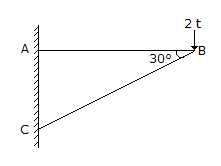A. 23t B. 32t C. 4 t D. 3 t.

Explanation:

No answer description available for this question. Let us discuss.

55.

A vehicle weighing w kg is to run on a circular curve of radius r. If the height of its centre of gravity above the road level is h and the distance between the centres of wheels is 2a, the maximum velocity, in order to avoid over turning, will be

 A.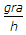B.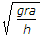C.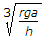D.